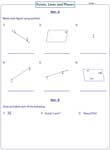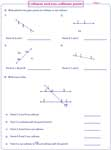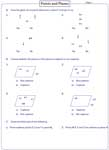1. Worksheets>
2. Math>
3. Geometry>
4. Points, Lines and Planes

Points, Lines and Planes Worksheets

The worksheets contain exercises to identify and draw the points, lines and planes. Exclusive worksheets on planes include collinear and coplanar concepts. Interesting descriptive charts, multiple choice questions and word problems are included in these worksheets.

Chart: Points, lines, rays and planes

Self-descriptive charts contain the definition, diagrammatic representation, symbolic representation and differences between a point, line, ray, line segment and a plane. Chart 3 describes the collinear and coplanar concepts.Identify points, lines and planes

In section A, identify the figure as a line, ray, line segment or a plane and represent them using their symbols. Draw them as described in section B.Collinear and non-collinear points

In part A, judge the position of points and find if the points are collinear or non-collinear. In part B, read the figure and declare the statements as true or false.Understanding plane

In part A, observe the set of points to determine a plane. In part B, answer the forced choice questions on coplanar concepts. In part C, draw the described figures.Questions based on figures

Read the given figure and answer all the word problems in these worksheets to become familiar with the concepts of points, lines and planes.Lines, Rays and Line Segments: Exclusive

It contains an enormous worksheets on identifying, naming and drawing lines, rays and line segments, simple word problems and printable charts.

Lines, Rays and Line Segments Worksheets

Obtain the entire collection of worksheets on points, lines and planes in a jiffy!

Related Worksheets